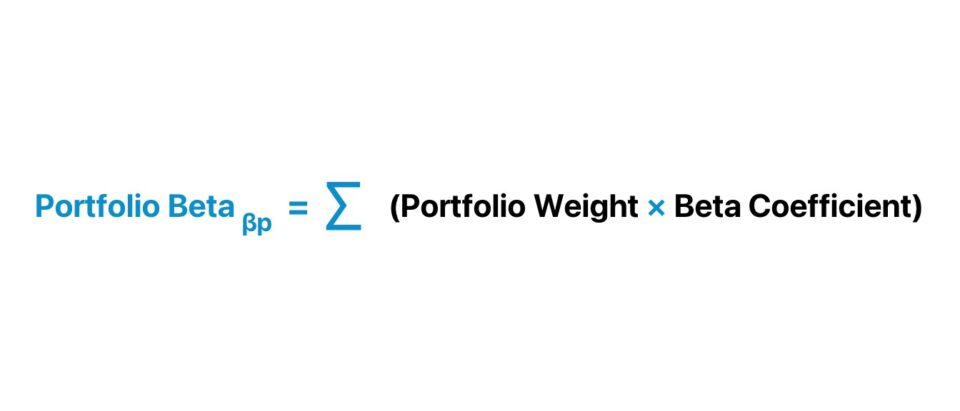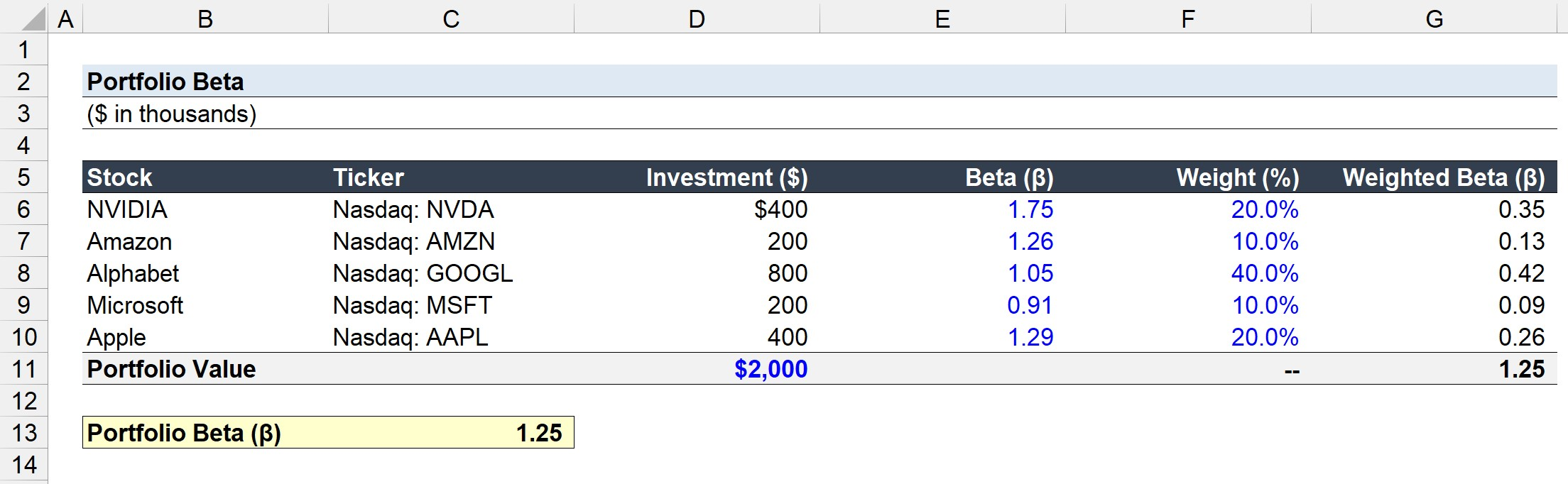Welcome to Wall Street Prep! Use code at checkout for 15% off.# Portfolio Beta

Guide to Understanding Portfolio Beta## How to Calculate Portfolio Beta?

The portfolio beta represents the overall systematic risk of a portfolio of securities.

Beta (β) is a measure of risk that reflects the sensitivity of an individual security or portfolio to market risk, i.e. the fluctuations in the prices of securities in the broader market.

Conceptually, the portfolio beta is the expected volatility in returns relative to the market as a whole.

Therefore, investors often analyze the portfolio beta to determine the expected return on their collection of investments to protect their returns against market risk.

In portfolio management, diversification is a critical part of constructing a portfolio capable of mitigating market risk, since the total risk is spread across a wide range of different securities, asset classes, and industries (or sectors).

The portfolio beta can be calculated using the following four-step process:

1. Identify Beta Coefficient → The first step is to identify the beta coefficient for each security in the investment portfolio, which can be retrieved via financial data platforms such as Bloomberg.
2. Calculate Portfolio Weights (%) → The next step is to compute the percent weight attributable to each security in the portfolio. The portfolio weight is calculated by dividing the market value of the investment at present by the total portfolio value.
3. Determine the Weighted Beta → From there, the beta of each individual security can be multiplied by its respective portfolio to arrive at each security’s weighted beta.
4. Portfolio Beta Calculation → In the final step, the sum of the weighted betas calculated thus far represents the portfolio beta.

## How to Interpret Portfolio Beta?

The general rules of thumb to interpret the portfolio beta are virtually identical to interpreting the beta of individual securities.

Because a higher beta coincides with undertaking more risk – i.e. the minimum rate of return expected by an investor increases – a rational investor should expect to earn a higher potential yield to compensate for the incremental risk.

So, what are the general rules for interpreting the beta of a portfolio?

Guidelines Description
Beta (β) = 1.0
• Sensitivity of the Investment Portfolio is Equivalent to the Market Benchmark
Beta (β) > 1.0
• Investment Portfolio is More Sensitive to Volatility Risk than the Market Benchmark
Beta (β) < 1.0
• Investment Portfolio is Less Sensitive to Volatility Risk than the Market Benchmark
Beta (β) < 0
• Inverse Relationship Between Portfolio Sensitivity and Market Benchmark (e.g. Gold)

If the beta on a portfolio is 0.5, the portfolio is anticipated to be half as volatile as the broader market.

If the stock market (S&P 500) were to rise by 10.0%, the portfolio should expect to increase in value by 5.0%.

Gold is a commodity that moves in an inverse direction to the stock market, i.e. with a negative beta.

Hence, market participants have historically flocked to gold as a “haven” amid periods of volatility and uncertainty surrounding the near-term economic outlook.

With that said, the price of gold should be expected to increase if the S&P 500 were to decline (or vice versa).

## Is Portfolio Beta Levered or Unlevered?

So, does the beta of each security need to be unlevered and relevered at the target capital structure?

The portfolio beta does not need to be unlevered because the metric reflects the risk of the portfolio in comparison to the market as a whole.

Why? The risks associated with the portfolio should include the effects of leverage, i.e. the percentage of debt in the capital structure.

The concept of unlevered beta is more common in corporate finance – namely, in a discounted cash flow (DCF) analysis – where the effects of a company’s capital structure (debt-equity mix) and underlying business risk must be removed, but that adjustment is not necessary here.

## Portfolio Beta Formula

Formulaically, the portfolio beta is the weighted sum of the beta coefficients of the securities in an investment portfolio.

Portfolio Beta = ∑ (Portfolio Weight × Beta Coefficient)

Since the portfolio weights of the securities are a proportion of the total portfolio, the sum must equal 1.0 (or 100%), or else a mistake had occurred.

## Portfolio Beta Calculator

We’ll now move to a modeling exercise, which you can access by filling out the form below.#### Excel TemplateSubmitting...

## Portfolio Beta Calculation Example

Suppose an investor is analyzing the beta of a tech-oriented portfolio to determine its risk profile and protect against potential losses.

On the date that the analysis was performed, the total portfolio value amounted to \$2 million.

• Total Portfolio Value = \$2 million

The investor’s portfolio is currently composed of the following securities.

Stock Ticker Investment (\$) Beta (β) Weight (%)
NVIDIA Corporation
• NASDAQ: NVDA
• \$400k
• 1.75
• 20.0%
Amazon.com
• NASDAQ: AMZN
• \$200k
• 1.26
• 10.0%
Alphabet Inc.
• NASDAQ: GOOGL
• \$800k
• 1.05
• 40.0%
Microsoft Corporation
• NASDAQ: MSFT
• \$200k
• 0.91
• 10.0%
Apple Inc.
• NASDAQ: AAPL
• \$400k
• 1.29
• 20.0%

The “Investment (\$)” is determined by multiplying each security’s “Weight %” assumption by the total portfolio value.

Investment (\$) = Portfolio Weight (%) × Portfolio Value

Using the “PRODUCT” function in Excel, we can calculate the weighted beta of the five securities.

=PRODUCT(Beta, Weight %)
• NVIDIA Corporation (NASDAQ: NVDA) = 1.75 × 20.0% = 0.35
• Amazon.com (NASDAQ: AMZN) = 1.26 × 10.0% = 0.13
• Alphabet Inc. (NASDAQ: GOOGL) = 1.05 × 40.0% = 0.42
• Microsoft Corporation (NASDAQ: MSFT) = 0.91 × 10.0% = 0.09
• Apple Inc. (NASDAQ: AAPL) = 1.29 × 20.0% = 0.26

The portfolio beta is the sum of the weighted beta of each security in the portfolio, which comes out to 1.25. Therefore, the portfolio is implied to carry substantial risk (i.e. more than the market overall) and is likely to fluctuate significantly in total value if the economy were to undergo an unexpected downturn or enter a long-term recessionary period.Step-by-Step Online Course

### Everything You Need To Master Financial Modeling

Enroll in The Premium Package: Learn Financial Statement Modeling, DCF, M&A, LBO and Comps. The same training program used at top investment banks.